Search datasheet (1.687.043 components) Search fieldPart namePart descriptionAbout site Manufacturers list ChipFindIC search engine AllXrefCross-reference database### Datasheet: AP20P02GJ (Advanced Power Electronics Corp.)

P-CHANNEL ENHANCEMENT MODE POWER MOSFET
Download:PDFZIP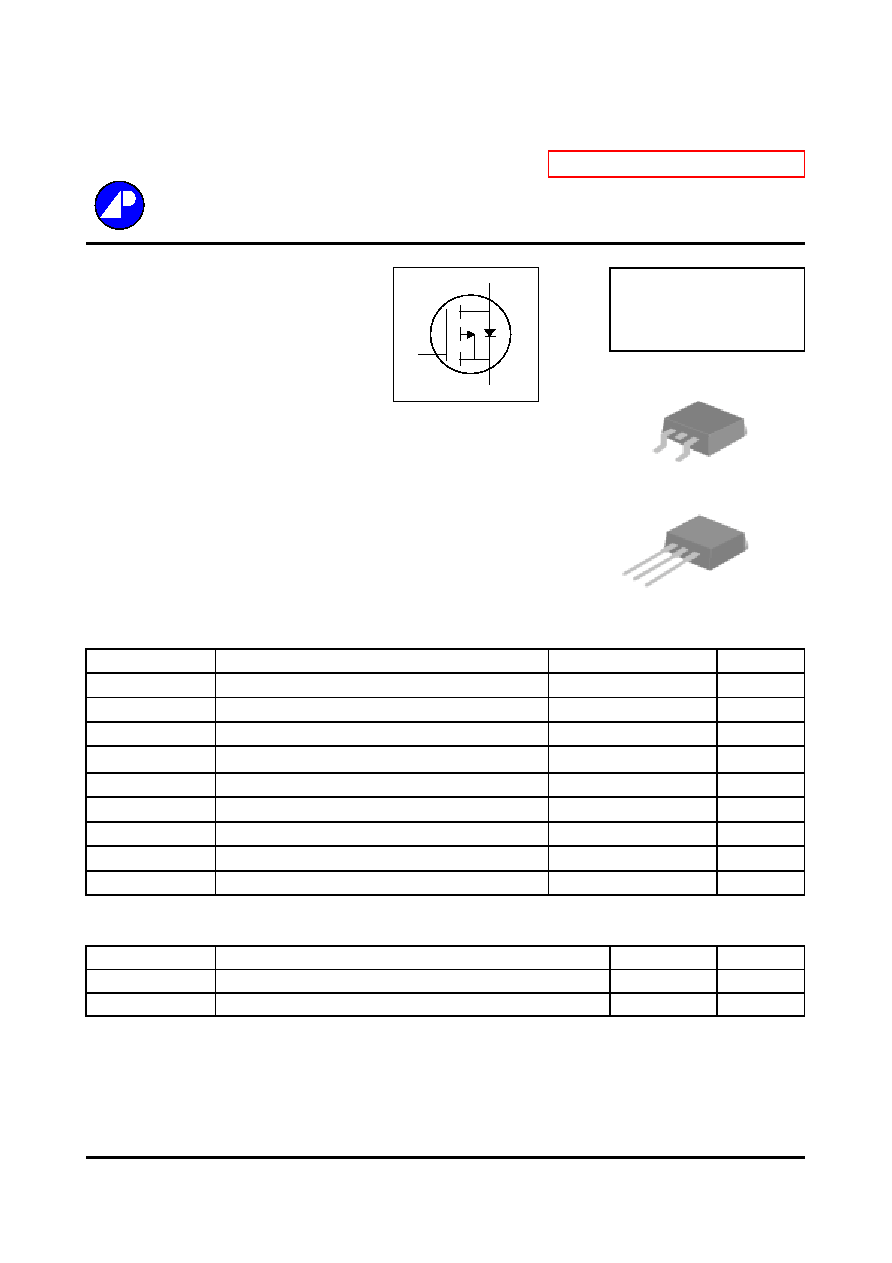P-CHANNEL ENHANCEMENT MODE
Electronics Corp.
POWER MOSFET
Simple Drive Requirement
BV
DSS
-20V
2.5V Gate Drive Capability
R
DS(ON)
52m
Fast Switching
I
D
-18A
Description
Absolute Maximum Ratings
Symbol
Units
V
DS
V
V
GS
V
I
D
@T
C
=25
A
I
D
@T
C
=100
A
I
DM
A
P
D
@T
C
=25
W
W/
T
STG
T
J
Symbol
Value
Unit
Rthj-c
Thermal Resistance Junction-case
Max.
4.0
/W
Rthj-a
Thermal Resistance Junction-ambient
Max.
110
/W
Data and specifications subject to change without notice
201225023
AP20P02GH/J
Rating
- 20
� 12
-18
0.25
Continuous Drain Current, V
GS
@ 10V
-14
Pulsed Drain Current
1
-50
Thermal Data
Parameter
Total Power Dissipation
Operating Junction Temperature Range
Storage Temperature Range
Pb Free Plating Product
-55 to 150
Linear Derating Factor
31.25
-55 to 150
Parameter
Drain-Source Voltage
Gate-Source Voltage
Continuous Drain Current, V
GS
@ 10V
G
D
S
G D
S
TO-252(H)
G
D
S
TO-251(J)
The Advanced Power MOSFETs from APEC provide the
designer with the best combination of fast switching,
ruggedized device design, low on-resistance and cost-effectiveness.
The TO-252 package is universally preferred for all commercial-
industrial surface mount applications and suited for low voltage
applications such as DC/DC converters. The through-hole version
(AP20P02GJ) are available for low-profile applications.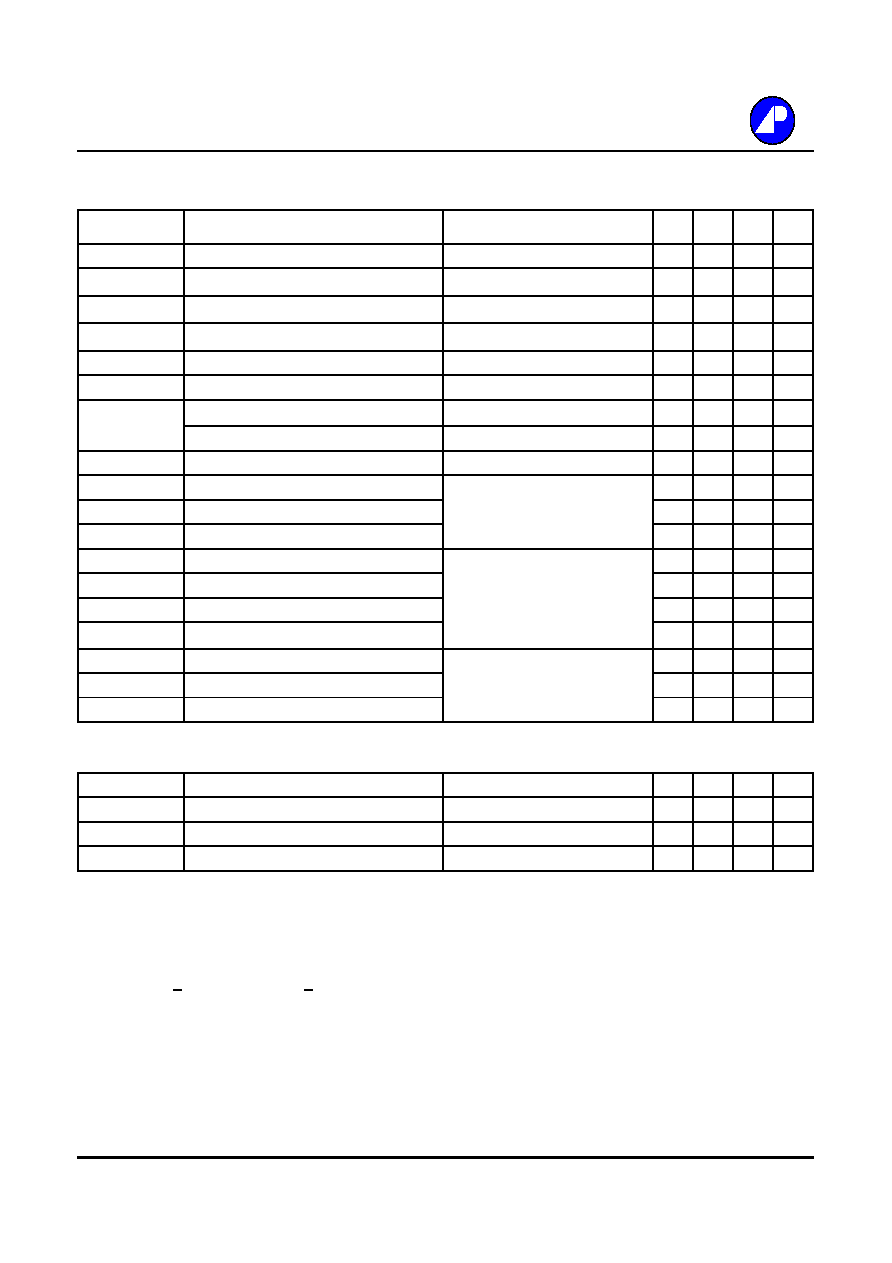AP20P02GH/J
Electrical Characteristics@T
j
=25
o
C(unless otherwise specified)
Symbol
Parameter
Test Conditions
Min. Typ. Max. Units
BV
DSS
Drain-Source Breakdown Voltage
V
GS
=0V, I
D
=-250uA
-20
-
-
V
B
V
DSS
/T
j
Breakdown Voltage Temperature Coefficient
Reference to 25
, I
D
=-1mA
-
-0.03
-
V/
R
DS(ON)
Static Drain-Source On-Resistance
V
GS
=-4.5V, I
D
=-8A
-
-
52
m
V
GS
=-2.5V, I
D
=-5A
-
-
85
m
V
GS(th)
Gate Threshold Voltage
V
DS
=V
GS
, I
D
=-250uA
-0.5
-
-
V
g
fs
Forward Transconductance
V
DS
=-10V, I
D
=-8A
-
15
-
S
I
DSS
Drain-Source Leakage Current (T
j
=25
o
C)
V
DS
=-20V, V
GS
=0V
-
-
-1
uA
Drain-Source Leakage Current (T
j
=150
o
C)
V
DS
=-16V, V
GS
=0V
-
-
-25
uA
I
GSS
Gate-Source Leakage
V
GS
= � 12
-
-
nA
Q
g
Total Gate Charge
2
I
D
=-8A
-
13.5
-
nC
Q
gs
Gate-Source Charge
V
DS
=-16V
-
2.1
-
nC
Q
gd
Gate-Drain ("Miller") Charge
V
GS
=-4.5V
-
1.6
-
nC
t
d(on)
Turn-on Delay Time
2
V
DS
=-10V
-
12
-
ns
t
r
Rise Time
I
D
=-8A
-
20
-
ns
t
d(off)
Turn-off Delay Time
R
G
=3.3
,
V
GS
=-4.5V
-
45
-
ns
t
f
Fall Time
R
D
=1.25
-
27
-
ns
C
iss
Input Capacitance
V
GS
=0V
-
1050
-
pF
C
oss
Output Capacitance
V
DS
=-16V
-
410
-
pF
C
Reverse Transfer Capacitance
f=1.0MHz
-
110
-
pF
Source-Drain Diode
Symbol
Parameter
Test Conditions
Min. Typ. Max. Units
I
S
Continuous Source Current ( Body Diode )
V
D
=V
G
=0V , V
S
=-1.2V
-
-
-10
A
I
SM
Pulsed Source Current ( Body Diode )
1
-
-
-50
A
V
SD
Forward On Voltage
2
T
j
=25
, I
S
=-10A, V
GS
=0V
-
-
-1.2
V
Notes:
1.Pulse width limited by safe operating area.
2.Pulse width <300us , duty cycle <2%.
100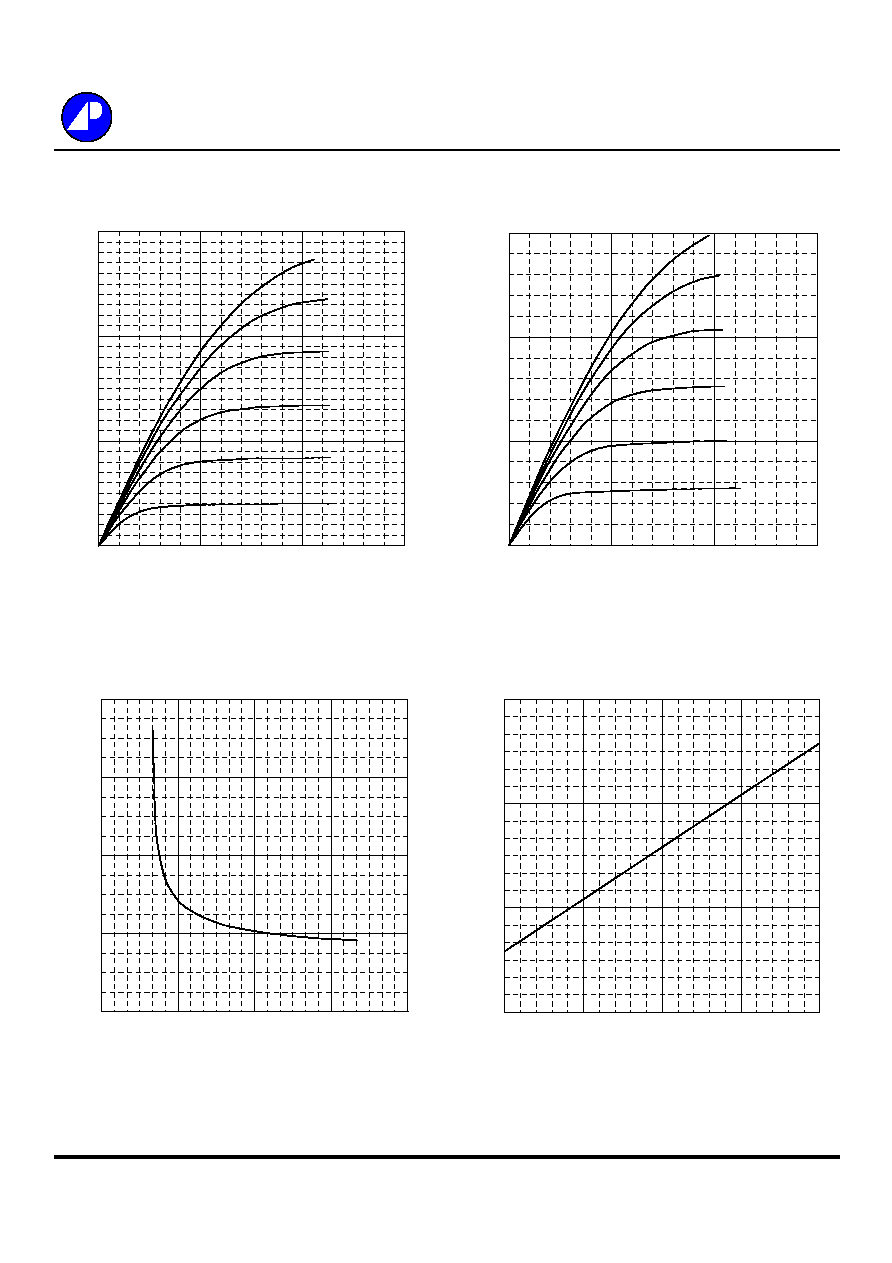Fig 1. Typical Output Characteristics
Fig 2. Typical Output Characteristics
Fig 3. On-Resistance v.s. Gate Voltage
Fig 4. Normalized On-Resistance
v.s. Junction Temperature
AP20P02GH/J
0
20
40
60
0
2
4
6
-V
DS
, Drain-to-Source Voltage (V)
-I
D
,
Dra
i
n C
u
rre
nt
(A
)
T
C
=25
o
C
-4.5V
-4.0V
-3.5V
-3.0V
-2.5V
V
GS
= -2.0V
0
15
30
45
0
2.5
5
7.5
-V
DS
, Drain-to-Source Voltage (V)
-I
D
, D
r
ain C
u
rrent (A
)
T
C
=150
o
C
-4.5V
-4.0V
-3.5V
-3.0V
-2.5V

V
GS
= -2.0V
0
40
80
120
160
0
3
6
9
12
-V
GS
(V)
R
DS
(
ON)
(m

)
I
D
= -8A
T
c
=25
0.2
0.8
1.4
2
-50
0
50
100
150
T
j
, Junction Temperature (
o
C)
No
rma
l
i
z
e
d
R
DS
(
ON)
I
D
= -8A
V
GS
= -4.5V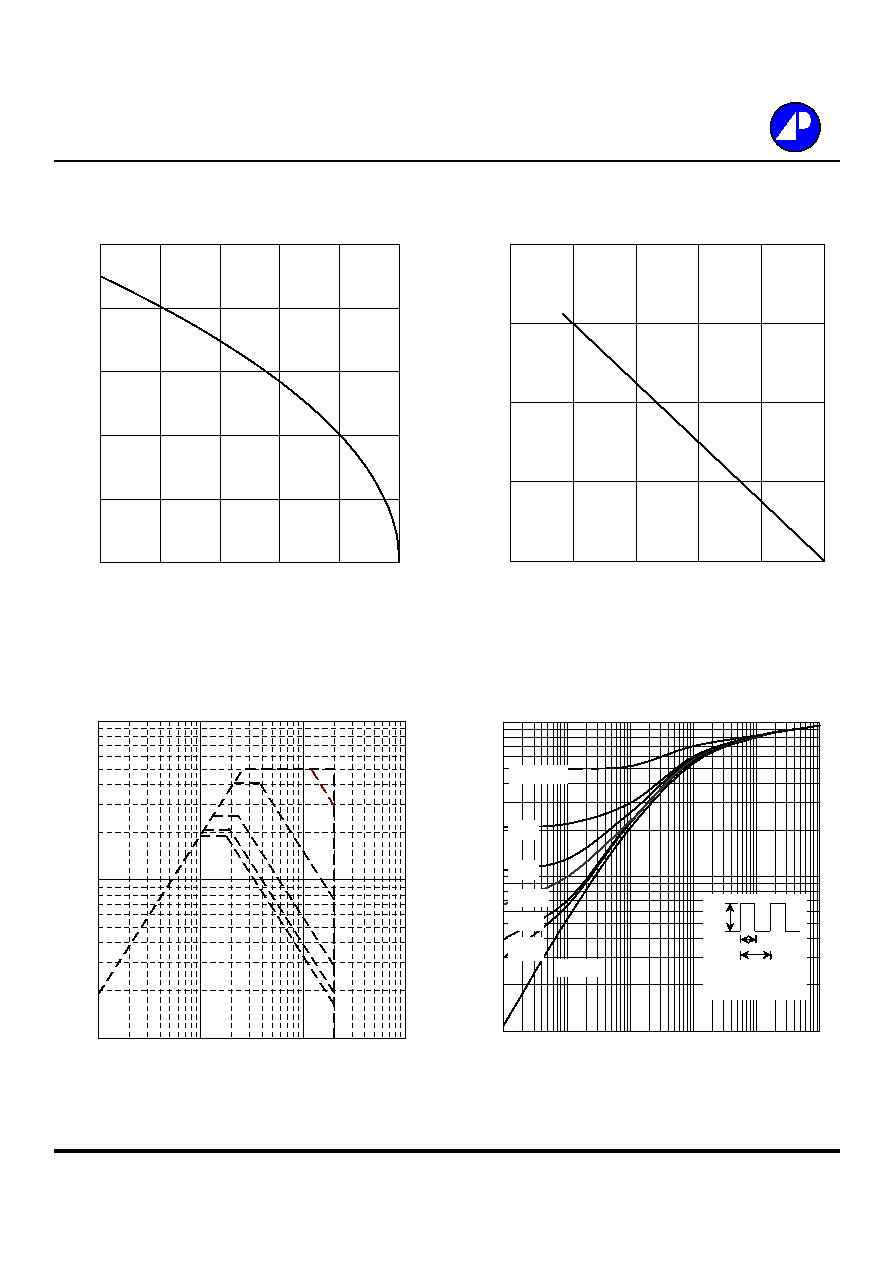AP20P02GH/J
Fig 5. Maximum Drain Current v.s.
Fig 6. Typical Power Dissipation
Case Temperature
Fig 7. Maximum Safe Operating Area
Fig 8. Effective Transient Thermal Impedance
0
4
8
12
16
20
25
50
75
100
125
150
T
c
, Case Temperature (
o
C)
-I
D
,
Dra
i
n C
u
rre
nt
(A
)
0
10
20
30
40
0
30
60
90
120
150
T
c
, Case Temperature (
o
C)
P
D
(W
)
0.01
0.1
1
0.00001
0.0001
0.001
0.01
0.1
1
t , Pulse Width (s)
N
o
r
m
aliz
ed Th
er
m
a
l Res
pon
s
e
(
R
th
jc
)
P
DM
Duty Factor = t/T
Peak T
j
= P
DM
x R
thjc
+ T
C
t
T
0.02
0.01
0.05
0.1
0.2
Duty Factor = 0.5
Single Pulse
1
10
100
0.1
1
10
100
-V
DS
(V)
-I
D
(A
)
T
C
=25 �C
Single Pulse
100us
1ms
10ms
100ms
DC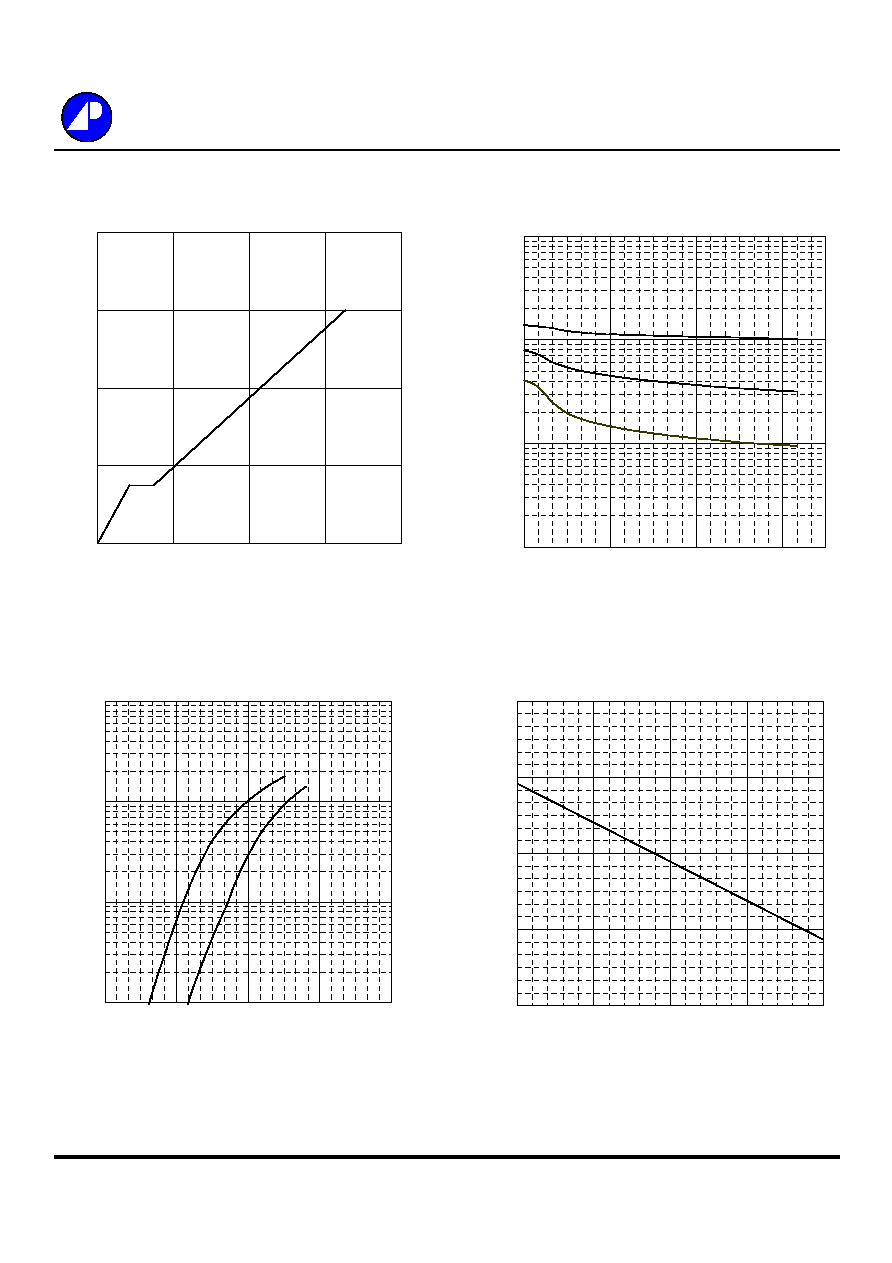Fig 9. Gate Charge Characteristics
Fig 10. Typical Capacitance Characteristics
Fig 11. Forward Characteristic of
Fig 12. Gate Threshold Voltage v.s.
Reverse Diode
Junction Temperature
AP20P02GH/J
0
2
4
6
8
0
5
10
15
20
Q
G
, Total Gate Charge (nC)
-V
GS
, G
a
te to S
o
u
r
ce Voltage (
V
)
I
D
= -8A
V
DS
= -16V
10
100
1000
10000
1
7
13
19
-V
DS
(V)
C (
p
F)
f=1.0MHz
Ciss
Coss
0
1
10
100
0.2
0.5
0.8
1.1
1.4
-V
SD
(V)
-I
S
(A
)
T
j
=25
o
C
T
j
=150
o
C
0
0.3
0.6
0.9
1.2
-50
0
50
100
150
T
j
, Junction Temperature (
o
C)
-V
GS(
t
h)
(V
)© 2020 • ICSheet• Contact form• Main page